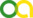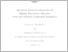(2019). Quantum geometrodynamics of higher derivative theories with and without conformal symmetry. PhD thesis, Universität zu Köln.Preview
PDF
doktroarbeitBN.pdf

Abstract

This thesis concerns with a framework of canonical quantization of gravity based on the EinsteinHilbert action extended by terms quadratic in curvature. The aim is to investigate the semiclassical limit of such a theory and compare it with the semiclassical limit of the canonical quantization of the Einstein-Hilbert action alone, the latter of which is the usual approach in this framework. General Relativity has passed the tests from the length scales of micrometers up to the cosmological scales. The classical evolution of our Universe seems to be described by the socalled ΛCDM model, which was recently tested by the Planck satelite with success. The recent discovery of gravitational waves seems to confirm also the linearized, long-range behavior of vacuum General Relativity. However, the behavior of gravity at short scales and relatively high energies, i.e. in the regimes where quantum effects of matter fields and spacetime become relevant, remains so far within the many possible theoretical approaches to its understanding. It is expected that near the initial singularity of our Universe - the Big Bang - the description of gravity drastically deviates from General Relativity and a theory of quantum gravity is necessary. But already near the theoretical limit of the highest observable energy scale (energy per excitation of a quantum field) - the Planck energy scale - it is expected that the effects of quantum field-theoretical description of matter propagating on classical curved spacetimes play a significant role. Because of this, General Relativity changes in two ways. First, the energy-momentum tensor is replaced by the expectation value of the energy-momentum tensor operator. Second, since the latter diverges, the regularization of these divergences has shown that it is necessary to modify General Relativity by adding to the Einstein-Hilbert action, among others, terms quadratic in curvature such as the square of the Ricci scalar and the square of the Weyl tensor. Since these terms generate fourth order derivatives in the modified Einstein equations, the doors were opened for investigating modified classical theories of gravity, in order to provide alternative interpretations of dark matter and the accelerated expansion of the Universe. However, an often neglected fact in these classical approaches is that these terms are suppressed at the present, classical scales. This is also reflected in the fact that the respective coupling constants of these new terms are proportional to the Planck constant and are thus of perturbative nature. Therefore they are only relevant at high energy/strong curvature regimes, typical for the very early universe. At extremely high energy scales, i.e. near and above the Planck energy scale, it is expected that the perturbative description breaks down and that a full quantum theory of gravity - which assumes that the spacetime itself is quantized as well - is necessary. The main goal of this thesis is to quantize the Einstein-Hilbert action extended by the quadratic curvature terms is within the canonical quantization approach, thus formulating quantum geometrodynamics of the higher derivative theories. The motivation is to provide an alternative to the standard canonical quantization based on the Einstein-Hilbert action alone, because the latter does not generate the quadratic curvature terms in the semiclassical limit. A particular formulation of a semiclassical approximation scheme is employed which ensures that the effects of the quadratic curvature terms become perturbative in the semiclassical limit. This leaves the classical General Relativity intact, while naturally giving rise to its first semiclassical corrections. Another topic of interest is a classical theory where the quadratic Ricci scalar and the Einstein-Hilbert term are absent from the action, which then enjoys the symmetry with respect to the conformal transformation of fields (local Weyl rescaling). We pay a special attention tothis case, because near and beyond Planck scales it is expected that conformal symmetry plays a very important role, since it provides a natural setting for the absence of the notion of a physical length scale. Certain useful model-independent tools are also constructed in this thesis. Firstly, it is shown that if coordinates are treated as dimensionless and if a set of variables based on the unimodular decomposition of the metric is introduced, the only conformally variant degree of freedom becomes apparent. This makes the geometrical origin of the physical length scale apparent as well, which is especially important in the interpretations of conformally invariant quantum theories of gravity. With such an approach several earlier results become much more transparent. Secondly - which naturally follows from the application of the set of these new variables - a model-independent generator of conformal field transformations is constructed in terms of which a reformulation of the definition of conformal invariance is given. Thirdly, it is argued that a canonical quantization scheme makes more sense to be based on the quantization of generators of relevant transformations, than on the first class constraints. The thesis thus attempts to combine several minor but important aspects of a theoretical approach and use them to pursue the main goal.

Item Type: Thesis (PhD thesis)
Creators:
CreatorsEmailORCIDORCID Put Code
Nikolic, BranislavUNSPECIFIEDorcid.org/0000-0003-0499-1166UNSPECIFIED
URN: urn:nbn:de:hbz:38-116950
Date: 2019
Language: English
Faculty: Faculty of Mathematics and Natural Sciences
Divisions: Faculty of Mathematics and Natural Sciences > Department of Physics > Institute for Theoretical Physics
Subjects: Natural sciences and mathematics
Physics
Uncontrolled Keywords:
KeywordsLanguage
quantum gravityEnglish
higher derivativesEnglish
general relativityEnglish
wheeler de witt equationEnglish
canonical quantizationEnglish
semi-classical approximationEnglish
Date of oral exam: 16 September 2019
Referee:
Kiefer, ClausProf. Dr.
Giulini, DomenicoProf. Dr.
Mazumdar, AnupamProf. Dr.
Refereed: Yes
URI: http://kups.ub.uni-koeln.de/id/eprint/11695View Item